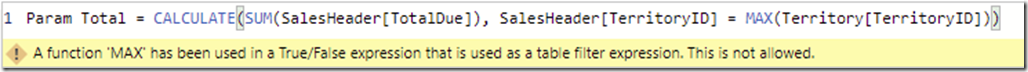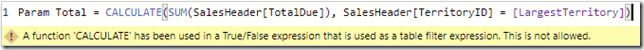# DAX error: A function ‘XXXX’ has been used in a True/False expression that is used as a table filter expression. This is not allowed.

Whenever you start trying to use more complicated filters in the CALCULATE or CALCULATETABLE functions in DAX, you may start to get the following error:

`A function 'MAX' has been used in a True/False expression that is used as a table filter expression. This is not allowed.`The function in single quotes may vary. Instead of MAX, it could be SUM, MIN, AVERAGE or nearly anything. Sometimes, you may not even be using a function and the error will just say CALCULATE is the problem:

`A function 'CALCULATE' has been used in a True/False expression that is used as a table filter expression. This is not allowed.`## What causes this error?

The error is caused by using a TRUE/FALSE expression, something that evaluates to TRUE or FALSE, to filter the table in a way that CALCULATE or CALCULATETABLE doesn’t support.  So the error is saying you can’t use a boolean comparison to filter your table except in very specific circumstances.

The following comparisons are not supported:

1. Comparing to a column to a measure. SalesHeader[TerritoryID] = [LargestTerritory]
2. Comparing a column to a an aggregate value. SalesHeader[TerritoryID] = MAX(TerritoryID[TerritoryID]])
3. Comparing a column to a What-If parameter. SalesHeader[TerritoryID] =

TerritoryParameter[TerritoryParameter Value]

In fact, you only have three options if you want to filter a column in a CALCULATE/CALCULATETABLE function:

1. Compare the column to a static value. SalesHeader[TerritoryID] = 6
2. Use variables to create a static value. VAR LargestTerritory = MAX(SalesHeader[TerritoryID])

This is because CALCULATE was designed for safety and performance. Complex row based comparisons can dramatically affect performance. So, in order to do more complex comparisons, you have to take the safety feature off and use the FILTER function.

## How do I fix it?

In order to fix the issue, wrap your expression in the FILTER function. To use the FILTER function, you need to pass in the table you want to filter, and then a TRUE/FALSE expression to determine which rows get return. So, let’s say we had the following code:

```CALCULATE (
)```

to use the FILTER function, we would use this:

```CALCULATE (